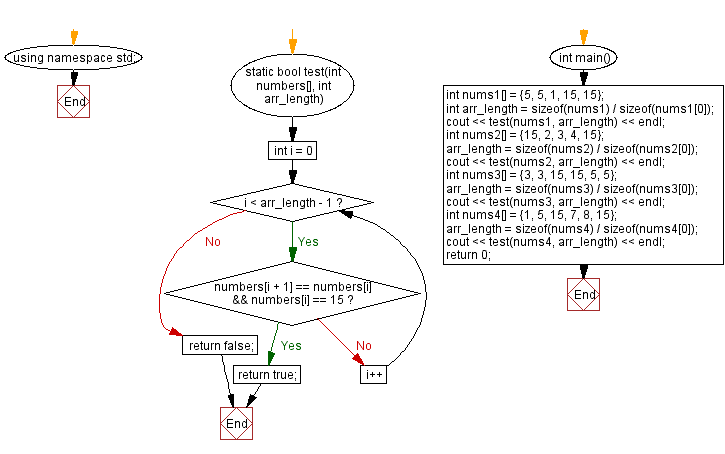﻿ C++ Exercises: Compare two given strings and return the number of the positions where they contain the same length 2 substring - w3resource# C++ Exercises: Compare two given strings and return the number of the positions where they contain the same length 2 substring

## C++ Basic Algorithm: Exercise-32 with Solution

Write a C++ program to compare two given strings and return the number of the positions where they contain the same length 2 substring.

Sample Solution:

C++ Code :

``````#include <iostream>

using namespace std;

int test(string str1, string str2)
{
int ctr = 0;
for (int i = 0; i < str1.length()-1; i++)
{
string firstString = str1.substr(i, 2);
for (int j = 0; j < str2.length()-1; j++)
{
string secondString = str2.substr(j, 2);
if (firstString==secondString)
ctr++;
}
}
return ctr;
}

int main()
{
cout << test("abcdefgh", "abijsklm") << endl;
cout << test("abcde", "osuefrcd") << endl;
cout << test("pqrstuvwx", "pqkdiewx") << endl;
return 0;
}
``````

Sample Output:

```1
1
2
```

Flowchart:C++ Code Editor:

Contribute your code and comments through Disqus.

What is the difficulty level of this exercise?

﻿